# 基于融合的夜间去雾算法研究Research of Nighttime Image Dehazing by Fusion

DOI: 10.12677/CSA.2018.85089, PDF, HTML, XML, 下载: 811  浏览: 3,070

Abstract: The outdoor images taken under the weather conditions such as fog and haze will result in degradation of contrast and color distortion. Due to the absorption and scattering of suspended particles, this degradation seriously affects the development of outdoor vision system. At present, there are few researches on image restoration for haze with uneven illumination. And the haze at night has the characteristics of low overall brightness, uneven illumination, color cast and noise. It’s difficult to dehaze. In this paper, a fusion based haze day image restoration framework is proposed based on the characteristics of haze day images (uneven illumination, low brightness, and blurred details). A new brightness adjustment curve is proposed for uneven illumination and low overall brightness. In order to ensure the enhancement of brightness and avoid exposure, the light map is generated. In detail, the light color of the object is relatively bright in the shallow depth of field; that is, the saturation is higher, but the brightness is low. With the increase of the depth of field, the fog in-creases, but the saturation decreases. Based on this, we derive a new transmission graph estimation method and generate detail maps. In the process of merging the above two images, three weights are used (the weight of the brightness, the weight of saturation, the weight of the saliency) to ensure that the better part of each image can be retained and the effect of fog removal can be achieved.

1. 引言

2. 光学模型

${I}_{i}^{\lambda }={L}_{i}{R}_{i}^{\lambda }t+{A}_{i}^{\lambda }\left(1-{t}_{i}\right)$ (1)

$i$$\lambda$ 分别表示像素的位置和RGB通道。 ${L}_{i}$ 表示入射光强度， ${R}_{i}^{\lambda }$ 为场景反射率， ${A}_{i}^{\lambda }$ 为局部大气光， $t={\text{e}}^{-\beta {d}_{i}}$ 为传输图， $\beta$ 表示大气衰减系数， ${d}_{i}$ 表场景与相机之间的距离。式(1)中第一项和第二项分别称为直接投射光和环境光。分描述目标与反射光的衰减过程和成像路径上周围杂散光的累积过程。在夜间条件下，由于光照不均匀，为消除不均匀光照的影响。假设在大气中介质分布是均匀的，改写式(1)的到式(2)

${\stackrel{^}{I}}_{i}^{\lambda }={R}_{i}^{\lambda }{t}_{i}+{\stackrel{^}{A}}^{\lambda }\left(1-{t}_{i}\right)$ (2)

3. 去雾算法

3.1. 算法框架

3.2. 输入图像生成

3.2.1. 亮度图生成

$\begin{array}{l}L\left(x\right)=x+sigma_s\cdot g\left(x\right)-sigma_h\cdot g\left(1-x\right)\\ g\left(x\right)={x}^{{c}_{1}}\mathrm{exp}\left(-{c}_{2}x\right)\end{array}$ (3)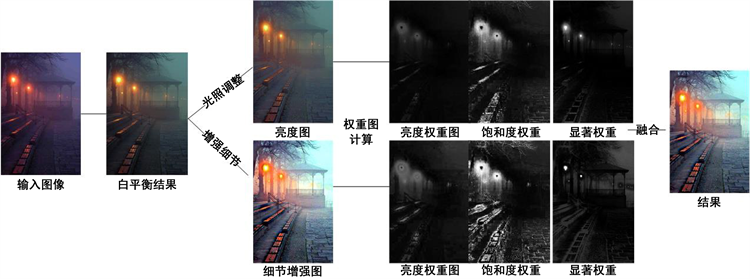Figure 1. This article algorithm flow chart

3.2.2. 细节图生成

${I}_{i}^{\lambda }={J}_{i}^{j}t+{A}_{i}^{\lambda }\left(1-{t}_{i}\right)$ ，在一个局部块 $\Omega$ ，根据上述观察，引入局部均值 $M\left(x,y\right)$ 根据大气光散射模型用 ${V}_{i}\left(x,y\right)$ 代替 ${A}_{i}\left(1-t\left(x,y\right)\right)$ ，然后改写退化模型。

${M}_{I}\left(x,y\right)={M}_{\text{J}}\left(x,y\right)t\left(x\right)+V\left(x,y\right)$ (4)

$V\left(x,y\right)=\mathrm{max}\left(\mathrm{min}\left(\rho B\left(x,y\right),{M}_{I}\left(x,y\right)\right),0\right)$ (5)

$\stackrel{¯}{M}\left(x,y\right)=\underset{\left(x,y\right)\in \Omega }{\mathrm{min}}\left(K\left({x}_{i},{y}_{j}\right)\right)$ (6)

$S\left({x}_{i},{y}_{j}\right)=\mathrm{max}\left({I}_{l}\left({x}_{i},{y}_{j}\right)\right)-\mathrm{min}\left({I}_{l}\left({x}_{i},{y}_{j}\right)\right)$ (7)

$t\left(x,y\right)=1-\frac{V\left(x,y\right)}{{A}_{\Omega }}$ (8)

$J\left(x,y\right)=\frac{I\left(x,y\right)-{A}_{\Omega }}{\mathrm{max}\left(t\left(x,y\right),\epsilon \right)}+{A}_{\Omega }$ (9)

3.3. 权重图Figure 2. Generation detail image intermediate results

${W}_{L}^{k}=\sqrt{1/3\left[{\left({R}^{k}-{L}^{k}\right)}^{2}+{\left({G}^{k}-{L}^{k}\right)}^{2}+{\left({B}^{k}-{L}^{k}\right)}^{2}\right]}$ (10)

${W}_{C}^{k}=\mathrm{exp}\left(-\frac{{\left({S}^{k}\left(x\right)-{S}_{\mathrm{max}}^{k}\right)}^{2}}{2{\sigma }^{2}}\right)$ (11)

$V\left(p\right)={\sum }_{\forall q\in I}G\left(p,q\right)$ (12)

$G\left(p,q\right)=|{I}_{p}-{I}_{q}|$ (13)

$V\left(p\right)=|{I}_{p}-{I}_{1}|+|{I}_{p}-{I}_{2}|+\cdot \cdot \cdot +|{I}_{p}-{I}_{M}|$ (14)

M为图像中像素点的个数，根据式(13)和式(14)，当像素点值相同时，它们的显著性值相同。以灰色图像为例，对于图像I中的强度 ${I}_{p}$ ，计算相对应的显著性值，式(15)。

$V\left({I}_{p}\right)={\sum }_{j=0}^{L-1}{N}_{j}G\left({I}_{p},j\right)$ (15)

$F\left(x\right)=\sum {\stackrel{¯}{W}}^{k}\left(x\right){I}_{k}\left(x\right)$ (16)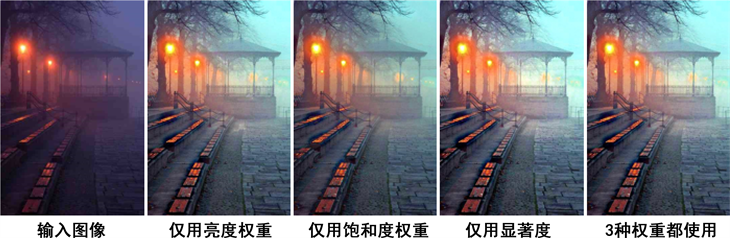Figure 3. Results using one weight map

4. 实验结果与分析

4.1. 本文算法结果

4.2. 主观评价分析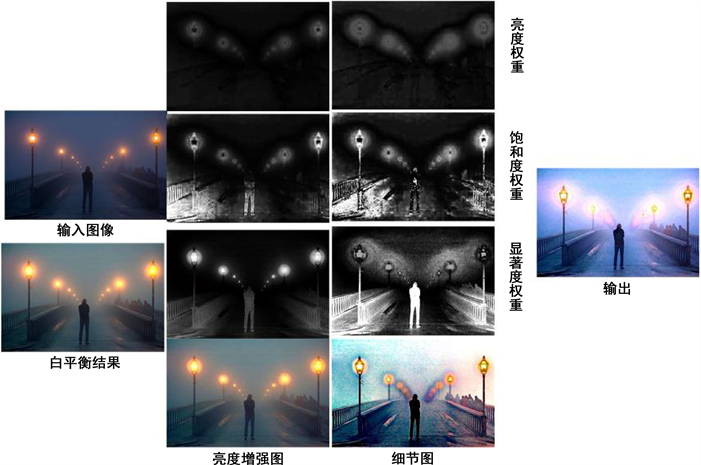Figure 4. This algorithm results and intermediate process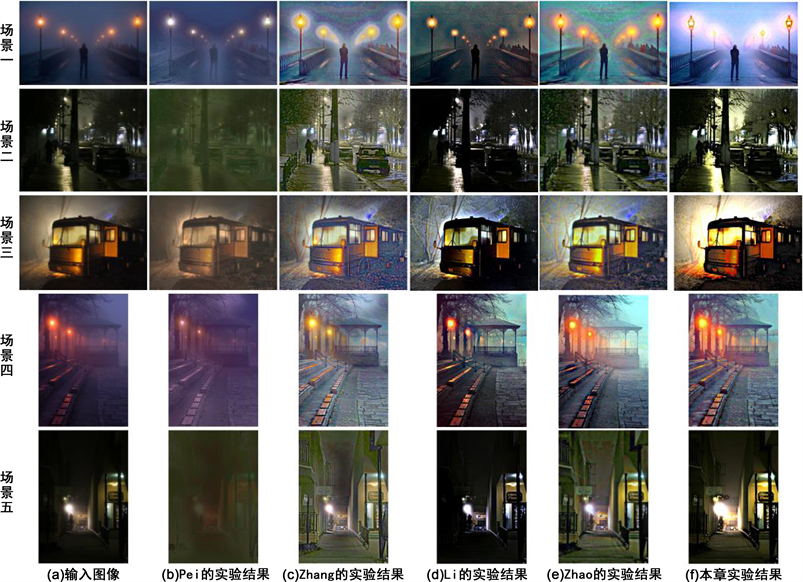Figure 5. Comparison with other algorithm

4.3. 客观评价分析

1) NESS梯度结构相似度。ChunLing Yang等  根据结构相似的思想结合人眼视觉系统的相关设计的。步骤如下：先为待评价图像制造参考图像。定义待评价图片I，参考图像使用 ${I}_{r}=LPF\left(I\right)$ ，即对待评价图像使用低通滤波得到参考图像。选择使用基于圆盘模型的均值滤波器和高斯模型的平滑滤波器都可以。为了更好的与成像系统匹配，建议使用 $7×7$ 高斯平滑滤波。但是在实际处理中使用同等大小的均值滤波器并不会使评价效果下降很大。之后提取带评价图像I和参考图像 ${I}_{r}$ 的梯度信息。利用人眼对水平方向和垂直方向的边缘最为敏感的特性，使用Sobel算子分别提取两幅图片的梯度图像 $G$${G}_{r}$ 。最后将图像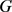${G}_{r}$ 划分成 $8×8$ 的小块，快间步长为4，即相邻重复率50%。计算每块的，方差越大表面梯度

$NRSS=1-\frac{1}{N}\underset{i=1}{\overset{N}{\sum }}SSIM\left({x}_{i},{y}_{i}\right)$ (17)

2) EAV点锐度。Ni. J等  提出一种基于边缘锐度算法来评价图像清晰度。通过统计图像某一方向的灰度变化来评价。公式入下：

$D\left(f\right)=\frac{{\sum }_{a}^{b}{\left(\text{d}f/\text{d}x\right)}^{2}}{|f\left(b\right)-f\left(a\right)|}$ (18)

$EAV=D\left(f\right)=\frac{\sum {}_{x,y}{\sum }_{i=1}^{8}|\text{d}f/\text{d}x|}{M×N}$ (19)

3) 基于自然场景统计的无参考图像质量评价算法(NIQE)。A. Mittal等  提出了基于自然场景统计的无参考图像质量评价算法，通过归一化的局部亮度系数提取多种可能反应图像质量的自然图像特征(文献  中详细介绍了所提取的特征)，并使用多元高斯模型(MVG)来拟合这些特征。文章中指出未退化图像的归一化亮度系数是符合高斯分布的，而退化图像(不管退化类型)不满足这种分布。所以，对于输入的评价图像，其MVG模型越接近自然图像的MVG模型，其图像的质量越高。自然图像的MVG模型是通过统计图像库中大量的自然为退化图像得到的。公式如下所示：

$D\left({v}_{1},{v}_{2},\sum ,\sum \right)=\sqrt{{\left({v}_{1}-{v}_{2}\right)}^{\text{T}}{\left(\frac{{\sum }_{1}+{\sum }_{2}}{2}\right)}^{-1}\left({v}_{1}-{v}_{2}\right)}$ (20)Table 1. Comparison with the NESS values of resultsTable 2. Comparison with the EVA values of resultsTable 3. Comparison with the NIQE values of results

5. 总结

  Pei, S.C. and Lee, T.Y. (2012) Nighttime Haze Removal Using Color Transfer Pre-Processing and Dark Channel Prior. Proceedings of the IEEE International Conference on Image Processing, Orlando, 30 September-3 October 2012, 957-960.  He, K., Sun, J. and Tang, X. (2011) Single Image Haze Removal Using Dark Channel Prior. IEEE Transactions on Pattern Analysis and Machine Intelli-gence, 33, 2341-2353. https://doi.org/10.1109/TPAMI.2010.168  Zhang, J., Cao, Y. and Wang, Z. (2014) Nighttime Haze Removal Based on a New Imaging Model. Proceedings of the IEEE International Conference on Image Processing, Paris, 15-19 June 2014, 4557-4561.  Land, E.H. and McCann, J.J. (1971) Lightness and Retinex Theory. Journal of the Optical Society of America, 61, 1-11. https://doi.org/10.1364/JOSA.61.000001  Li, Y., Tan, R.T. and Brown, M.S. (2015) Nighttime Haze Removal with Glow and Multiple Light Colors. Proceedings of the IEEE International Conference on Computer Vision, Santiago, 11-18 De-cember 2015, 226-234.  Li, Y. and Brown, M. (2014) Single Image Layer Separation Using Relative Smoothness. Proceedings of the IEEE Conference on Computer Vision and Pattern Recognition, Columbus, 23-28 June 2014, 2752-2759.  Park, D., Han, D.K. and Ko, H. (2016) Nighttime Image Dehazing with Local Atmospheric Light and Weighted Entropy. IEEE International Conference on Image Processing, 25-28 September 2016, 2261-2265.  He, K., Sun, J. and Tang, X. (2013) Guided Image Filtering. IEEE Transactions on Pattern Analysis & Machine Intelligence, 35, 1397-1409. https://doi.org/10.1109/TPAMI.2012.213  Zhao, J., Feng, H., Xu, Z., et al. (2013) Detail Enhanced Multi-Source Fusion Using Visual Weight Map Extraction Based on Multi Scale Edge Preserving Decomposition. Optics Communications, 287, 45-52.  方帅, 赵育坤, 李心科, 等. 基于光照估计的夜间图像去雾[J]. 电子学报, 2016, 44(11): 2569-2575.  Chen, G.H., Yang, C.L. and Xie, S.L. (2007) Gradient-Base Structural Similarity for Image Quality Assessment. IEEE International Conference on Image Processing, 2, 2.  Ni, J. (2009) Image Sharpness Function Based on Edge Feature. Proceedings of SPIE, The International Society for Optical Engineering, 7513, 75131A-75131A-6.  Mittal, A., Soundararajan, R. and Bovik, A.C. (2013) Making a Completely Blind Image Quality Analyzer. IEEE on Signal Processing Letters, 22, 209-212. https://doi.org/10.1109/LSP.2012.2227726  Mittal, A., Moorthy, A.K. and Bovik, A.C. (2012) No-Reference Image Quality Assessment in the Spatial Domain. IEEE Transactions on Image Processing, 21, No. 12. https://doi.org/10.1109/TIP.2012.2214050